# RRB NTPC 2021 Exam Phase-2 Memory Based Maths Questions with Answers: Check Mathematics Questions asked in RRB NTPC 2021 CBT

RRB NTPC 2021 Exam Phase-2 Memory Based Maths Questions with Answers: Get the memory based questions from Mathematics Section that came in RRB NTPC 2020-21 Phase-2 Exam held in online mode from 16th Jan to 30th Jan 2021.

Created On: Jan 28, 2021 17:09 ISTRRB NTPC 2021 Exam Phase-2 Memory Based Maths Questions with Answers: Check Mathematics Questions asked in RRB NTPC 2021 CBT

RRB NTPC 2021 Exam Phase-2 Memory Based Maths Questions with Answers: RRB NTPC 2021 Phase-2 CBT Exam has been commenced from 16th January 2021 and will be conducted till 30th January 2021 for around 27 lakh Candidates across different exam centres in India. RRB NTPC 2021 Phase-3 will be conducted from 31st Jan to 12th Feb 2021 for around 28 Lakh candidates. Computer Based Test (CBT) is being conducted for the recruitment of RRB Non-Technical Popular Categories (NTPC) 35281 Vacancies (Graduate & Under-Graduate Posts) in two shifts.

In this article, we are going to share the important memory based General Intelligence & Mathematics section Questions as per the feedback received by the candidates who have appeared for RRB NTPC 2021 Phase-2 CBT Exam. Candidates are advised to definitely cover these questions for scoring high marks in the Exam. Let’s have a look at the Important Questions that are being covered in the RRB NTPC 2021 Exam:

 RRB NTPC 2021 Mathematics Section Memory Based Questions with Answer:

1. X alone can do a piece of work in 12 days and Y alone in 16 days. X and Y undertook to do it for Rs.6400. With the help of Z, they completed the work in 6 days. How much is to be paid to Z?

a) Rs. 375

b) Rs. 400

c) Rs. 600

d) Rs. 800

Answer: (b)

Explanation: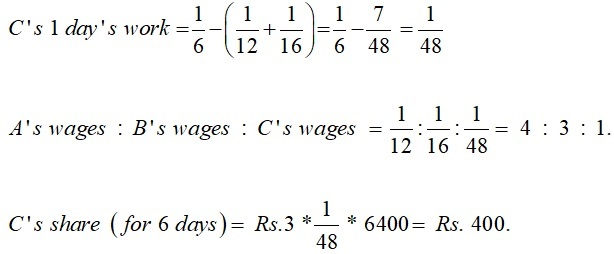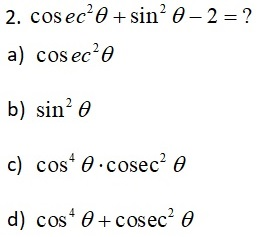Answer: (c)

Explanation: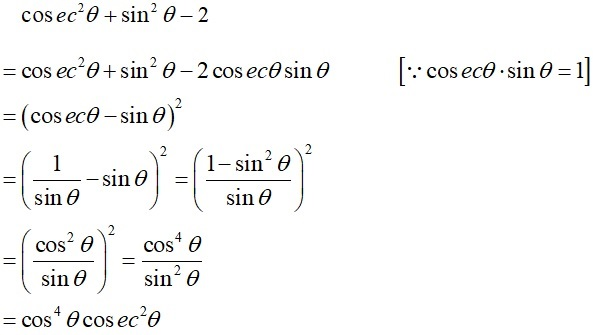3. Find out the ratio of length and the area of a rectangle if the ratio of length & perimeter of rectangle is 1:6 and the area of rectangle is 800 sq.m?

a) 1:20

b) 2:10

c) 5:11

d) 2:13

Answer: (a)

Explanation: Let the length is x & perimeter be 6x

Let the breadth be B

Then 2(x + B) = 6x

x + B = 3x
B = 2x

Now

Length × breadth = area

x × 2x = 800

2x2 = 800

x = 20

Hence length = 20 m

Ratio of length: area

20 : 400

1 : 20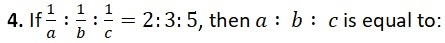a) 5 : 3 : 2

b) 6 : 10 : 15

c) 2 : 3 : 5

d) 15 : 10 : 6

Answer: (d)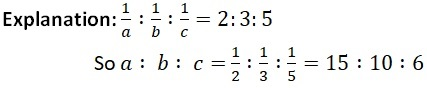5. A started a business investing Rs. 50,000 in 2011. In 2012, he invested an additional amount of Rs. 20,000 and B joined him with an amount of Rs. 70,000. In 2013, B invested another additional amount of Rs. 20,000 and C joined them with an amount of Rs. 70,000. What will be A’s share in the profit of Rs. 2, 10,000 earned at the end of 3 years from the start of the business in 2011?

a) Rs. 1,00,000

b) Rs. 95,000

c) Rs. 1,20,000

d) None of these

Answer: (b)

Explanation: Ratio of A, B and C.

A: B: C = (50000 X 12 + 70000 X 12 + 70000 X 12) : (70000 X 12 + 90000) : (70000 X 12)

= 2280000: 1920000: 840000 =19: 16: 7

A’s share = Rs. (2, 10,000 × 19/42) = Rs. 95,000

6. P and Q can do a piece of work in 45 and 40 days respectively. They began the work together but P leaves after some days and Q finished the remaining work in 23 days. Then P has worked for:

a) 9

b) 21

c) 32

d) None of these

Answer: (a)

Explanation: Work done by P in one day =1/45 and by Q = 1/40

Work done by both in days = 8 + 9/360 = 17/360

Work done by Q = 9 23 = 207,

Remaining work is done by P & Q = 360 – 207/17 = 9 days.

7. If x - (1/x) = 3, then value of x3 – (1/x3) is:

a) 32

b) 36

c) 40

d) 49

Answer: (b)

Explanation: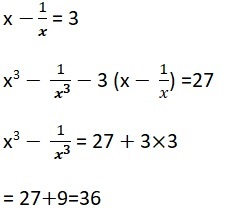8. (0.16)7/ (0.064)3 × (0.4)2=(0.4)?

a) 5

b) 6

c) 7

d) 8

e) None of the above

Answer: c)

Explanation: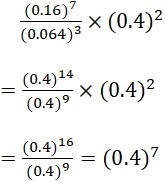9. X, Y and Z start a business. X invests one-third of the capital, Y invests One-fourth of the capital and the rest is invested by Z. The share of Z in the total profit of Rs. 480000 is.

a) Rs. 160000

b) Rs. 180000

c) Rs. 240000

d) Rs. 200000

Answer: d)

Explanation:  X : Y : Z = 1/3 : 1/4 : 5/12 = 4: 3: 5

Z’s share = 480000 X 5/12 = 200000

10. In an examination, 34% of the students failed in mathematics and 42% failed in English. If 20% of the students failed in both the subjects, then find the percentage of students who passed in both the subjects.

a) 40%

b) 41%

c) 43%

d) 44%

Answer: d)

Explanation: Failed in mathematics, n (1) = 34

Failed in English, n (2) = 42

n (AUB) = n(1) + n (2) – n (A∩ B)

= 34 + 42- 20 = 56

Failed in either or both subjects are 56

Percentage passed = (100−56)% = 44%

Comment ()

8 + 8 =
Post